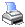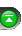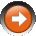NinjaScript > Language Reference > Indicator Methods > Relative Spread Strength (RSS)Description

Developed by Ian Copsey, Relative Spread Strength is a variation to the Relative Strength Index.

Syntax

 RSS(int eMA1, int eMA2, int length) RSS(IDataSeries input, int eMA1, int eMA2, int length)   Returns default value RSS(int eMA1, int eMA2, int length)[int barsAgo] RSS(IDataSeries input, int eMA1, int eMA2, int length)[int barsAgo]

Return Value

double; Accessing this method via an index value [int barsAgo] returns the indicator value of the referenced bar.

Parameters

 eMA1 First EMA's period eMA2 Second EMA's period input Indicator source data (?) length Number of bars used in the calculation

Examples

 // Prints the current value of the RSS using default price type double value = RSS(10, 40, 5); Print("The current RSS value is " + value.ToString());   // Prints the current value of the RSS using high price type double value = RSS(High, 10, 40, 5); Print("The current RSS value is " + value.ToString());

Source Code

You can view this indicator method source code by selecting the menu Tools > Edit NinjaScript > Indicator within the NinjaTrader Control Center window.